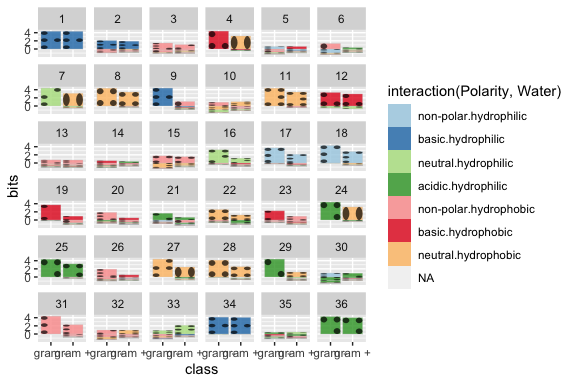# Creating a new alphabet for use with gglogo

#### 2020-01-28

By default, the gglogo package uses polygons created from a Helvetica font to use for creating logo plots. These polygons are stored in the object alphabet.rda, and use the name of the letter or digits they describe as their group identifier:

library(ggplot2)
library(gglogo)
data(alphabet)
head(alphabet)
##           x           y order group pathGroup
## 1 0.1208333 0.004854369     1     A       A.1
## 2 0.1229167 0.007281553     2     A       A.1
## 3 0.4437500 1.000000000     3     A       A.1
## 4 0.5729167 1.000000000     4     A       A.1
## 5 0.8833333 0.000000000     5     A       A.1
## 6 0.7604167 0.000000000     6     A       A.1

Each letter and digit is scaled to an area between 0 and 1 in y direction (which makes the lower case letter ‘c’ as tall as its upper case counterpart). In x direction the letter scaled proportionally to its extent in y direction.

ggplot(data = alphabet, aes(x = x, y = y)) +
geom_polygon() +
facet_wrap(~group, ncol = 13) +
coord_equal()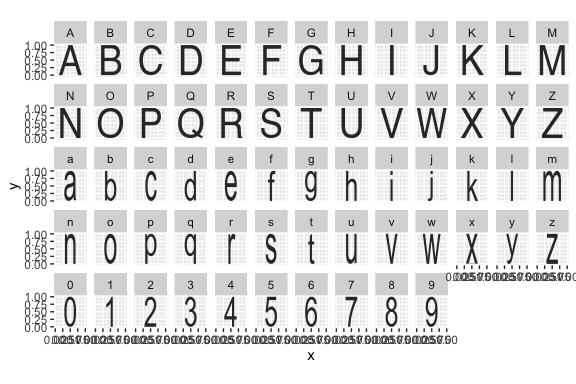In order to change the alphabet used, an alphabet object has to be created, and the link to the object has to be changed so that the logo plots use the correct alphabet in the rendering process.

# Creating a new alphabet

The function createPolygons have parameters to choose the font family and the set of letters for which polygons are supposed to be created. The parameters dim and fontsize are only needed, if some letters appear to be chopped off. In that case, the dimension of the image should be increased and/or fontsize should be decreased.

alphas <- c(LETTERS, letters, 0:9)
alphabet_garamond <- createPolygons(alphas, font="Garamond")
alphabet_garamond$group <- factor(alphabet_garamond$group, levels=alphas)

ggplot(alphabet_garamond, aes(x = x, y = y, group = group)) +
geom_polygon(aes(subgroup=pathGroup)) +
facet_wrap(~group, ncol = 13)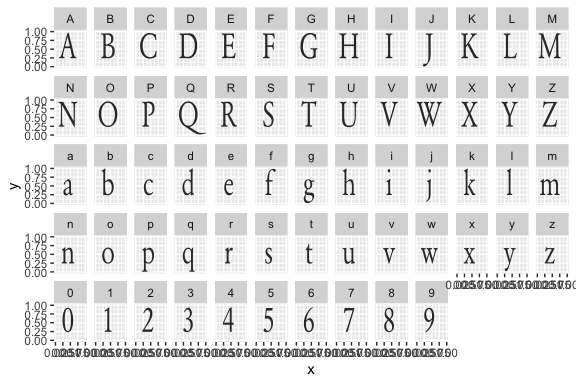alphas <- c(LETTERS, letters, 0:9)
alphabet_helvetica <- createPolygons(alphas, font="Helvetica")
alphabet_helvetica$group <- factor(alphabet_helvetica$group, levels=alphas)

ggplot(alphabet_helvetica, aes(x = x, y = y)) +
geom_polygon() +
facet_wrap(~group, ncol = 13)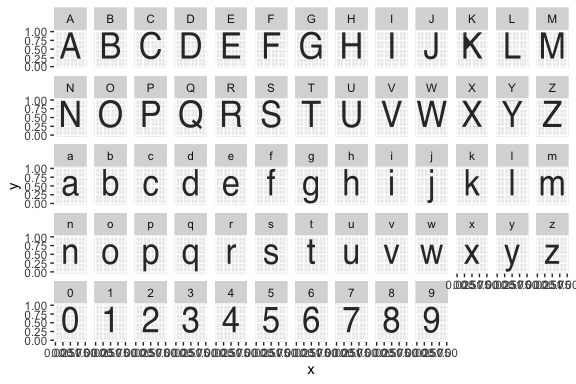For use as alphabet in the logo geom, letters have to be scaled to fill the full extent in y-direction so as to fill the surrounding rectangles. Setting the parameter scale to TRUE regulates this.

alphas <- c(LETTERS, letters, 0:9)
alphabet_comic <- createPolygons(alphas, font="Comic Sans MS", scale=TRUE)
alphabet_comic$group <- factor(alphabet_comic$group, levels=alphas)

ggplot(alphabet_comic, aes(x = x, y = y)) +
geom_polygon() +
facet_wrap(~group, ncol = 13)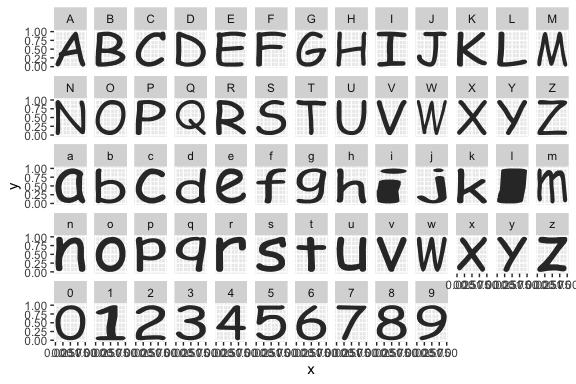Logo plot of peptide sequences, first with the unscaled Helvetica alphabet:

data(sequences)
ggplot(data = ggfortify(sequences, peptide, treatment = class)) +
geom_logo( aes(x = class, y = bits, label=element, group=element,
fill = interaction(Polarity, Water)),
alphabet = alphabet_braille,
alpha = 0.8) +
facet_wrap(~position) +
scale_fill_brewer(palette="Paired")
## Warning: cols is now required.
## Please use cols = c(position, element)